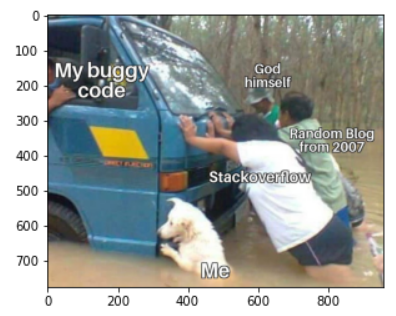# OpenCV入门### OpenCV

OpenCV是一个开源库，最初由Intel开发，它包含了支持计算机视觉和机器学习的便捷方法和函数。

import cv2
import numpy as np
import matplotlib.pyplot as plt

image = cv2.imread('img.jpg')
cv2.imshow('Some title', image)

cv2.waitKey(0)
cv2.destroyAllWindows()waitkey和.destroyAllWindows方法对于在不崩溃的情况下运行代码至关重要。

image = cv2.imread('img.jpg')
plt.imshow(image)OpenCV将图像加载为Numpy数组，并且它们具有三个维度：红色，绿色和蓝色。维度通常称为通道，它们保存的值介于0到255之间，代表每个像素的颜色强度。

>>> print(type(image))
>>> print(image.shape)

<class 'numpy.ndarray'>
(776, 960, 3)

Matplotlib使用RGB，这就是为什么我们的图片看起来很奇怪。这不是问题，因为OpenCV有一些非常方便的方法来转换颜色。

image = cv2.imread('img.jpg')
image = cv2.cvtColor(image, cv2.COLOR_BGR2RGB)

plt.imshow(image)### 色彩

def show(img):
fig, ax = plt.subplots(1, figsize=(12,8))
ax.axis('off')
plt.imshow(img, cmap='Greys')

image = cv2.imread('img2.jpeg')
image = cv2.cvtColor(image, cv2.COLOR_BGR2RGB)

show(image)image = cv2.imread('img2.jpeg')

gray = cv2.cvtColor(image, cv2.COLOR_BGR2GRAY)
image = cv2.cvtColor(gray, cv2.COLOR_GRAY2RGB)

show(image)img = cv2.imread('img2.jpeg')

B, G, R = cv2.split(img)
img = cv2.merge([B*0, G, R*0])

img = cv2.cvtColor(img, cv2.COLOR_BGR2RGB)
show(img)img = cv2.merge([np.ones_like(B)*255, G, R])

img = cv2.cvtColor(img, cv2.COLOR_BGR2RGB)
show(img)img = cv2.imread('img2.jpeg')
img = cv2.cvtColor(img, cv2.COLOR_BGR2HSV)

H, S, V = cv2.split(img)

img = cv2.merge([np.ones_like(H)*30, S+10, V-20])

img = cv2.cvtColor(img, cv2.COLOR_HSV2RGB)
show(img)HSV：色调、饱和度和明度。# read img and convert to HSV
img = cv2.cvtColor(img, cv2.COLOR_BGR2HSV)
# split dimensions
H, S, V = cv2.split(img)
# upper and lower boundaries
lower = np.array([80, 0, 0])
upper = np.array([120, 255, 255])
# assemble image
img = cv2.merge([H, S, V])
# convert to RGB and display
img = cv2.cvtColor(img, cv2.COLOR_HSV2RGB)
show(img)img = cv2.imread('img2.jpeg')
B, G, R = cv2.split(img)
show(B)

img = cv2.cvtColor(img, cv2.COLOR_BGR2HSV)
H, S, V = cv2.split(img)
show(S)#### 资源：

http://panchuang.net/

sklearn机器学习中文官方文档：
http://sklearn123.com/

http://docs.panchuang.net/